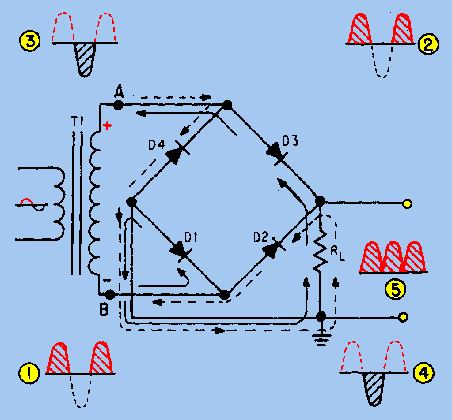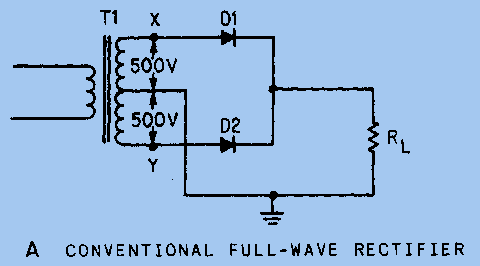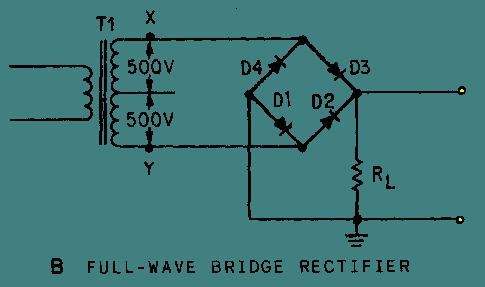Custom SearchThe Bridge Rectifier When four diodes are connected as shown in figure 4-8, the circuit is called a BRIDGE RECTIFIER. The input to the circuit is applied to the diagonally opposite corners of the network, and the output is taken from the remaining two corners. Figure 4-8. - Bridge rectifier.One complete cycle of operation will be discussed to help you understand how this circuit works. We have discussed transformers in previous modules in the NEETS series and will not go into their characteristics at this time. Let us assume the transformer is working properly and there is a positive potential at point A and a negative potential at point B. The positive potential at point A will forward bias D3 and reverse bias D4. The negative potential at point B will forward bias D1 and reverse bias D2. At this time D3 and D1 are forward biased and will allow current flow to pass through them; D4 and D2 are reverse biased and will block current flow. The path for current flow is from point B through D1, up through RL, through D3, through the secondary of the transformer back to point B. This path is indicated by the solid arrows. Waveforms (1) and (2) can be observed across D1 and D3. One-half cycle later the polarity across the secondary of the transformer reverses, forward biasing D2 and D4 and reverse biasing D1 and D3. Current flow will now be from point A through D4, up through RL, through D2, through the secondary of T1, and back to point A. This path is indicated by the broken arrows. Waveforms (3) and (4) can be observed across D2 and D4. You should have noted that the current flow through RL is always in the same direction. In flowing through RL this current develops a voltage corresponding to that shown in waveform (5). Since current flows through the load (RL) during both half cycles of the applied voltage, this bridge rectifier is a full-wave rectifier. One advantage of a bridge rectifier over a conventional full-wave rectifier is that with a given transformer the bridge rectifier produces a voltage output that is nearly twice that of the conventional full-wave circuit. This may be shown by assigning values to some of the components shown in views A and B of figure 4-9. Assume that the same transformer is used in both circuits. The peak voltage developed between points X and Y is 1000 volts in both circuits. In the conventional full-wave circuit shown in view A, the peak voltage from the center tap to either X or Y is 500 volts. Since only one diode can conduct at any instant, the maximum voltage that can be rectified at any instant is 500 volts. Therefore, the maximum voltage that appears across the load resistor is nearly - but never exceeds - 500 volts, as a result of the small voltage drop across the diode. In the bridge rectifier shown in view B, the maximum voltage that can be rectified is the full secondary voltage, which is 1000 volts. Therefore, the peak output voltage across the load resistor is nearly 1000 volts. With both circuits using the same transformer, the bridge rectifier circuit produces a higher output voltage than the conventional full-wave rectifier circuit. Figure 4-9A. - Comparison of a conventional and bridge full-wave rectifier. CONVENTIONAL FULL-WAVE RECTIFIERFigure 4-9B. - Comparison of a conventional and bridge full-wave rectifier. FULL-WAVE BRIDGE RECTIFIERQ.11 What is the main disadvantage of a conventional full-wave rectifier?Q.12 What main advantage does a bridge rectifier have over a conventional full-wave rectifier?Privacy Statement - Copyright Information. - Contact Us